Guide to Arrays in Python

In this tutorial, we will be exploring how to create an array in the Python programming language using the array module.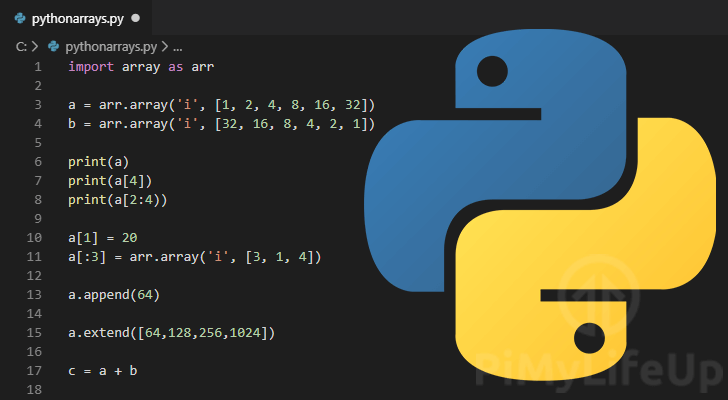Python does not have support for a traditional array as you would see in a programming language like “C” without importing a particular package.

By default, to have array-like support in Python, you can make use of Python lists or collections.

The only real difference between a list and an array in Python is that a list has no constraints on the data type.

With a list, you can use different data types without worry. Whereas with a “C” like array, the data type is constrained and can only be used with a single data type.

Typically you are better off using a Python list over a Python array as lists are handled faster and are a bit more versatile. However, they can be helpful if you ever need to interface with C code that is type strict.

Even though Python might not have built-in support for arrays, we can implement them using the array Python Module.

This tutorial will show you how to make use of the array module in Python to have a constrained implementation of arrays.

Python Array Tutorial Index

If you would like to skip to a certain section in this Python array guide, feel free to use the index below.

Comparision of Lists and Arrays in Python

To show you the difference between using lists and using the array module, we are going to show you two different code snippets.

The second snippet will intentionally throw an error to showcase the difference between the implementations.

Python List Example

This first code snippet shows you what a Python list looks like.

a = ["raspberry", 3.14, 4]

As you can see with this code snippet, we can use any data type we want within a list without any worries.

Python Array Example

This second snippet will show you what happens if you try to do the same thing with the array module.

import array as arr
a = arr.array('d', "raspberry", 3.14, 4)

If you try to run the code above, you will run into an error.

The reason for this is that two of the values are a different data type to the expected “double” data type.

Over the next several sections, we will show you how to put this array module to use in Python.

Creating Arrays in Python

1. To start creating an array, you need to import the array module.

import array as arr

Throughout our tutorial, we will be importing the array Python module using the as keyword.

We do this so that we only need to use arr to access the module.

2. With the array module imported, let’s now put it to use.

To create an array, you will need to decide what data type you want it to be constrained to.

Using the table below, you can see the type code for each of the possible data types.

List of Supported Type Codes for Python Arrays

Type CodeC Data TypePython Data TypeMinimum Size in Bytes
'b'signed charint1
'B'unsigned charint1
'h'signed shortint2
'H'unsigned shortint2
'i'signed intint2
'I'unsigned intint2
'l'signed longint4
'L'unsigned longint4
'q'signed long longint8
'Q'unsigned long longint8
'f'floatfloat4
'd'doublefloat8

3. We can create an array by using the array modules .array() function.

variable = arr.array(TYPE_CODE, [ARRAY])

The first argument defines the data type that you want to use in your array. For a list of the possible type codes, use our table above.

The second argument defines the array itself. The array is defined by square brackets ([ ]), with each element separated by a comma (,).

Below we have an example of creating an array in Python and assigning it to a Python variable called a.

Example on How to Create an Array

1. For this example, we are going to assume that you want an array that only accepts integers.

Using our table from earlier, we can see that we need to use the type code 'i'.

Within our integer constrained array, we are going to store six values (1, 2, 4, 8, 16, and 32), each value is multiplied by two to work out the next number.

a = arr.array('i', [1, 2, 4, 8, 16, 32])

2. Let’s now put this together so you can see what a simple Python array script looks like.

This script imports the array module, then creates an array stored in the variable a and prints out its value.

import array as arr

a = arr.array('i', [1, 2, 4, 8, 16, 32])
print(a)

If you were to run this small snippet, you would get the following output.

array('i', [1, 2, 4, 8, 16, 32])

You can see the data type is an array, the first argument defines the data type stored in it, and the second argument shows us the array itself.

Accessing Array Elements in Python

Accessing the elements of an array is a straightforward process that only requires you to know the index of the element that you are after in your Python array.

To access elements in an array, you need to make use of the index operator. The index operator is indicated by the square brackets, [ ] next to the array name.

arrayName[INDEX POSITION]

With an array, you have to imagine each separate value as being in an individually numbered cell with the first cell always being index 0.

This number is what we call an arrays index.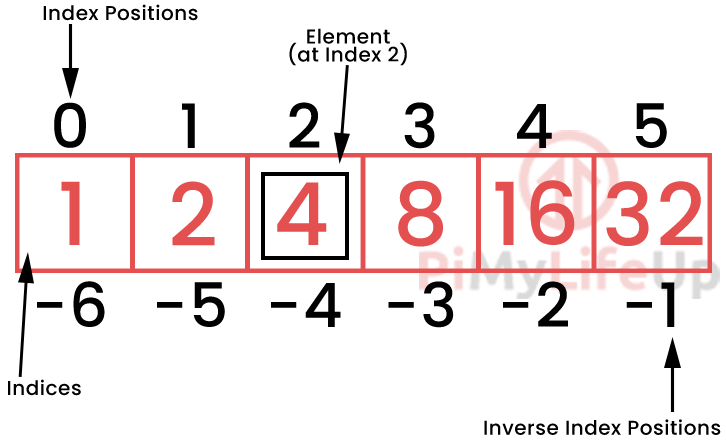Python also has what you could call its “inverse index positions“. Using this, you can read an array in reverse.

For example, if you use the index -1, you will be interacting with the last element in the array.

Knowing this, you can easily access each element of an array by using its index number.

For instance, if we wanted to access the number 16 in our array, all we need to do is use our variable called a with square brackets ([]) and the index position of the element.

Example of Accessing Elements in a Python array

Like most of the examples in this tutorial, we are going to start with this basic sample array.

import array as arr

a = arr.array('i', [1, 2, 4, 8, 16, 32])

So in this example, we are going to use the index position 4 to access the number 16 as it is the 4th element in the array.

print(a)

The main thing you have to remember when accessing elements in an array is that the index always starts from 0, not 1.

Slicing Arrays in Python

Using Python, it is possible to select a range of elements from an array by using the slice operator (:).

[START POSITION]:[END POSITION]

One thing to note is that the end index position is never included as part of the result of a slice.

For example, if you use [1:5], you are effectively saying 1 to 5, where the data in index position 5 is not included in the result.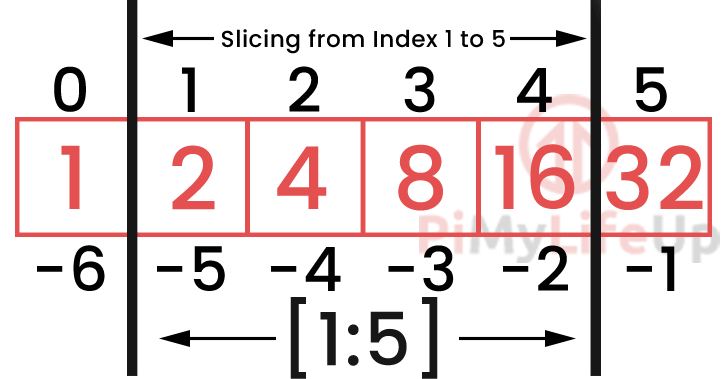To show how the slice operator works in Python we are going to use our trusty sample array.

import array as arr

a = arr.array('i', [1, 2, 4, 8, 16, 32])

Below we are going to run through three of the different ways that the slice operator can be used.

Basic Usage of the Slice Operator

The most basic usage of the slice operator is to use it just by itself within the square brackets.

print(a[:]) #Beginning to end

When used by itself, the slice operator will just output the entire array as it has no beginning or end point.

array('i', [1, 2, 4, 8, 16, 32])

Slicing an Array from the Beginning to an End Index

You can also use the slice operator to slice from the beginning of an array to an end index position.

:[END POSITION]

To do this, all you need to know is the index position you want to read to and add it to the right-hand side of the slice operator (:).

Example Code

For example, if we only wanted to grab the first three elements of the array, we can use the following.

print(a[:3])#Beginning to index position 3

Running this in Python, you can see that only the first three elements (0, 1, and 2 positions) are displayed.

array('i', [1, 2, 4])

In this result, you can see that the value at our end index position (Index 3) is not included in this output.

Slicing an Array from a Start Index to the End

You are also able to slice an array from a start index position to the end.

[START POSITION]:

Slicing from one position to the end is easy as specifying the start index on the left-hand side of the slice operator (:).

Example Code

For example, if we wanted to grab all elements after the 3rd element, we would use the slice operator with 3 on the left-hand side ([3:]).

print(a[3:])

From this bit of Python code, you should end up with the following result.

array('i', [8, 16, 32])

As you can see, we have every element in our array, excluding the first three elements (Array index 0 to 2).

Slicing the Middle of an Array

Slicing the middle of an array in Python is also pretty straightforward.

[START POSITION]:[END POSITION]

To slice a specific range in the array, all you need to do is specify both a start index position and an end index position while using the slice (:) operator.

Example Code

Using this is relatively simple. Let’s say that we wanted to grab the middle two elements of our array.

Our start index for this example will be 2, and our end index would be 4. These two indexes will get us the middle two elements of our array.

print(a[2:4))

Now, go ahead and run this little snippet.

From this snippet, you should see that the middle two elements of our sample array are returned (4 and 8).

array('i', [4, 8])

Changing Array Elements in Python

As arrays are mutable objects we can modify the individual elementsIn Python, it is even possible to change multiple elements of an array at once by using the slice operator.

For the examples in this section, we are going to be starting each of them with the following code snippet.

import array as arr

a = arr.array('i', [1, 2, 4, 8, 16, 32])

Changing Single Array Elements

The first thing we will learn about changing arrays is how to modify a single element.

Modifying a single element is as simple as knowing its position in the array and the value you want to assign to it.

arrayName[ARRAY INDEX] = NEW VALUE

As you can see, changing a single element is a very straightforward process and relies on you knowing the index position of the element that you want to modify.

Example of Changing Individual Elements in an Array

To put this into practice, we are going to use our example array and change the second element (Index position 1) to the number 20.

a = 20

As you can see in the snippet above, all we needed to do to change the second element is to use its index position, the equals (=) symbol, then finally the value we want to assign to it.

We can check to see that the value has changed by using the following code to print the value of our array.

print(a)

You then should be able to see the array with the single element we modified.

array('i', [1, 20, 4, 8, 16, 32])

Changing Multiple Array Elements

In Python, it is also possible to change multiple elements in an array at once.

To do this, you will need to make use of the slice operator and assign the sliced values a new array to replace them.

arrayName[STARTPOS:ENDPOS] = arr.array('TYPE_CODE', [NEW ARRAY])

The array you use must contain the same number of elements and be of the same type as the elements that you want to replace.

Example of Changing Multiple Elements in an Array

To give you an example of how to do this, we are going to replace the first three elements of our sample array with the numbers 3, 1, and 4.

For us to select the first three elements we will be using the slice operator with an end index of 3, for example [:3]

We then need to assign that slice a new array, which we can create easily by using the steps we learned earlier in this Python array tutorial.

a[:3] = arr.array('i', [3, 1, 4])

This little snippet will replace the first three elements with our new data.

You can verify this worked by printing out the value of our sample array with the following Python code.

print(a)

From this, you should now see the array with our newly replaced elements.

a[:3] = arr.array('i', [3, 1, 4])

Adding Elements to an Array in Python

To be able to add elements to an array in Python, we need to make use of two different functions.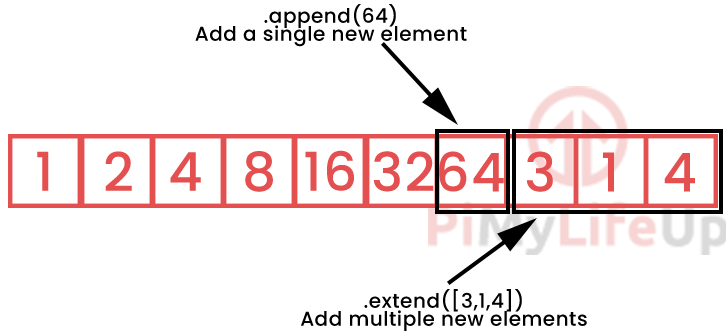The first of these functions is called .append(NEW ELEMENT). This function can be used to add a single new element to the end of an array.

The second of these functions is called .extend([NEW ELEMENTS]). You can use this function when you want to add multiple new elements to the end of an array.

Like all sections of this guide, we are going to be starting all of the following sections using our trusty sample code.

import array as arr

a = arr.array('i', [1, 2, 4, 8, 16, 32])

Adding an Element using the append() Function

Adding an element using the append() function is a fairly straightforward process.

.append(ELEMENT TO INSERT)

You call the append() function on any of your already created arrays. The function takes a single argument that is the element you want to add.

Example of Using the append() Function

Using the append() function is relatively simple. All you need is an array that already exists, which in our case is our a variable.

To this variable, we are going to add the number 64, which we can do thanks to the append function.

a.append(64)

You can see that the new value has been added by using the print() function on our a variable.

print(a)

You should now be able to see how the array looks with the additional element added.

array('i', [1, 2, 4, 8, 16, 32, 64])

Adding Elements using the extend() Function

If you want to add multiple elements at once, you will end up needing to use the extend() function.

arrayName.extend([LIST OF ELEMENTS])

Like the .append() function, this function is also a part of the array object. This means you will be using this with an already created array.

Example of Using the extend() Function

We can use the extend() function quite easily. All we need to do is provide it with a list of new elements to add.

For this example, we are going to continue our sample arrays pattern and add the following four numbers: 64, 128, 256, and 1024.

a.extend([64,128,256,1024])

As you can see, extending an array is as simple as using the correct function and passing in a list of all the values you want to add.

Just make sure these values are of the same data type as the originals.

We can check out what our a array looks like now by using the print() function.

print(a)

You should now see the extended version of the array.

array('i', [1, 2, 4, 16, 24, 48, 64, 128, 256, 1024])

Concatenating Two Arrays in Python

In Python, it is also possible to join two arrays together.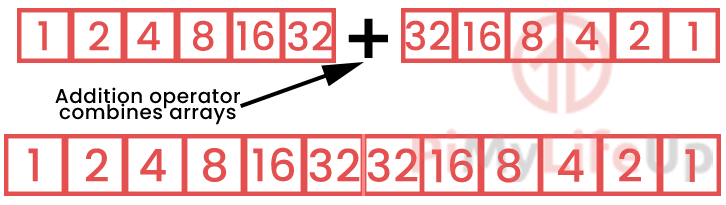To join two arrays together, all you need to do is use the addition operator +.

array1+array2

To showcase how to concatenate two arrays in Python, you can check out our example below.

Example of Concatenating two Arrays

In this example, we are going to make use of our usual sample array called a alongside one that is the reverse of it that we will call b.

import array as arr

a = arr.array('i', [1, 2, 4, 4, 16, 32])
b = arr.array('i', [32, 16, 8, 4, 2, 1])

We will be joining these two arrays together and storing them in a variable called c.

So that we can check out the result of the combination of our two arrays we will print the value of our new variable.

c = a + b
print(c)

As you can see from the result, combining two arrays is incredibly easy.

array('i', [1, 2, 4, 8, 16, 32, 32, 16, 8, 4, 2, 1])

Removing Elements from an Array

In this section, we will be showing you how to remove elements from an array.

To remove elements from an array in Python, we can make use of two different functions.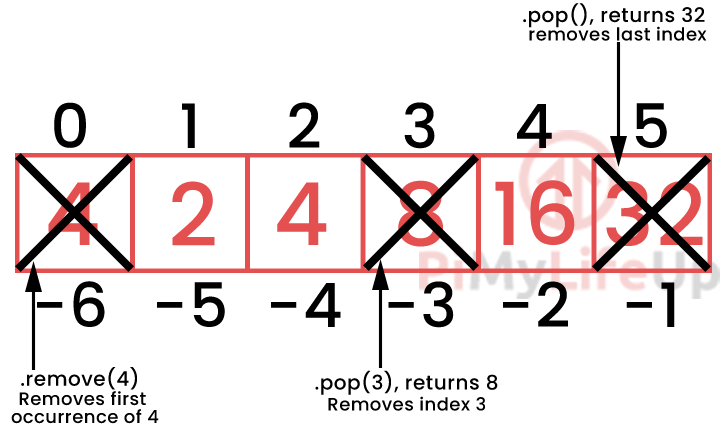The first of these functions is .remove(). This function is used to remove the first occurrence of an item with the specified value.

arrayName.remove(ITEMTOREMOVE)

The second function is .pop(). This function will return the value of the index that it is removing.

value = arrayName.pop(INDEXTOREMOVE)

For the following examples, we will be starting with the following code.

import array as arr

a = arr.array('i', [4, 2, 4, 8, 16, 32])

This snippet gives us enough code to demonstrate how the two functions work in Python.

The .remove() Array Function

Using the .remove() function is quite simple.

All you need to know is the value that you are looking to remove from your array.

arrayName.remove(ELEMENTDATATOREMOVE)

This function will automatically search your array for the value and remove the first occurrence.

Example of Using the .remove() Array Function

To showcase this behavior, we are going to go ahead and use the .remove() function on our array to remove the number 4 and print out the modified array.

a.remove(4)
print(a)

From the result of this, you should see that the first 4 value in the array was removed, but the second 4 still exists.

array('i', [2, 4, 8, 16, 32])

If you want to remove all occurrences of a certain value from your array, you will need to either run this command until it can no longer be found or use a function like filter().

The .pop() array Function

The .pop() function has some handy behaviors. Whenever you remove a certain index, its value is returned by the function.

value = arrayName.pop(ELEMENTINDEX)

The argument for this function is optional. If you don’t specify an index, -1 will be used.

Using  -1 will mean that the pop function will remove the last element in the array.

The way the .pop() function returns the value of the element it is removing can be useful if you want to process the data stored in it.

Example of Using the .pop() Array Function

Using our sample array, we will be showing you how the function works with, and without an argument.

Let’s start by using the .pop() function to remove the second element in our array.

We will be using the print() function to observe the values returned by .pop()

print(a.pop(1))
print(a)

Looking at the result of this we can observe two things.

The first thing is that the value of our second element is returned. The second thing is that our array is now missing the element that was “popped”.

2
array('i', [4, 4, 8, 16, 32])

Now, if we used the .pop() function again, but this time without an argument, you can see that it removes the last element in the array.

print(a.pop())
print(a)

Now again, let us check out the result from these two functions.

32
array('i', [4, 4, 8, 16])

As you can see, the last element in our Python array was removed, and its value of 32 was returned.

Hopefully, at this point, you will now have an understanding of how to use arrays in Python.

If you have run into any issues or have any feedback, feel free to drop a comment below.